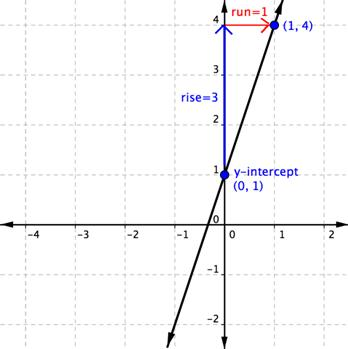## Writing Equations Using Point-Slope

### Learning Outcomes

• Write the equation of a line using slope and a point on the line
• Write the equation of a line using two points on the line

## Find the Equation of a Line Given the Slope and a Point on the Line

Using the slope-intercept equation of a line is possible when you know both the slope (m) and the $y$-intercept (b), but what if you know the slope and just any point on the line, not specifically the $y$-intercept? Can you still write the equation? The answer is yes, but you will need to put in a little more thought and work than you did previously.

Recall that a point is an $(x, y)$ coordinate pair and that all points on the line will satisfy the linear equation. So, if you have a point on the line, it must be a solution to the equation. Although you don’t know the exact equation yet, you know that you can express the line in slope-intercept form, $y=mx+b$.

You do know the slope (m), but you just don’t know the value of the y-intercept (b). Since point  $(x, y)$ is a solution to the equation, you can substitute its coordinates for $x$ and $y$ in $y=mx+b$ and solve to find b!

This may seem a bit confusing with all the variables, but an example with an actual slope and a point will help to clarify.

### Example

Write the equation of the line that has a slope of $3$ and contains the point $(1,4)$.

To confirm our algebra, you can check by graphing the equation $y=3x+1$. The equation checks because when graphed it passes through the point $(1,4)$.Write the equation of the line that has a slope of $\frac{7}{8}$ and contains the point $\left(4,\frac{5}{4}\right)$.

Watch the video below for another example of how to find the equation given the slope and a point on the line.

## Find the Equation of a Line Given Two Points on the Line

Let’s suppose you don’t know either the slope or the $y$-intercept, but you do know the location of two points on the line. It is more challenging, but you can find the equation of the line that would pass through those two points. You will again use slope-intercept form to help you.

The slope of a linear equation is always the same, no matter which two points you use to find the slope. Since you have two points, you can use those points to find the slope (m). Now you have the slope and a point on the line! You can now substitute values for $m, x$, and $y$ into the equation $y=mx+b$ and find b.

### Example

Write the equation of the line that passes through the points $(2,1)$ and $(−1,−5)$.

Notice that is doesn’t matter which point you use when you substitute and solve for b—you get the same result for b either way. In the example above, you substituted the coordinates of the point $(2, 1)$ in the equation $y=2x+b$. Let’s start with the same equation, $y=2x+b$, but substitute in $(−1,−5)$:

$\begin{array}{l}\,\,\,\,\,y=2x+b\\-5=2\left(-1\right)+b\\-5=-2+b\\-3=b\end{array}$

The final equation is the same: $y=2x–3$.

Write the equation of the line that passes through the points $(-4.6,6.45)$ and $(1.15,7.6)$.